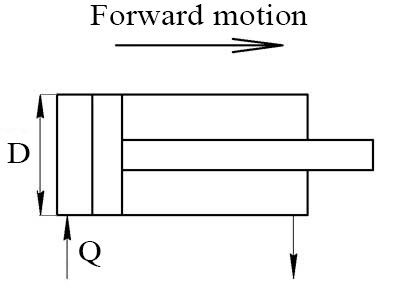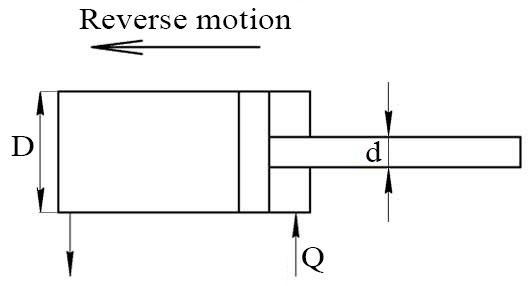# Hydraulic Cylinder Velocity Calculator

The velocity at which the piston rod of a hydraulic cylinder moves is based on the following equation:

V=Q/Ae
• V - velocity rate of piston rod
• Q - flow
• Ae - effective area

## Extended speed of cylinderOil flow Q: Units m3/s m3/min m3/h gpm lpm in3/min Piston diameter D: Units mm Millimetre cm Centimeter dm Decimeter m Meter km Kilometer in Inch

## Retracted speed of cylinderOil flow Q: Units m3/s m3/min m3/h gpm lpm in3/min Piston diameter D: Rod diameter d: Units mm Millimetre cm Centimeter dm Decimeter m Meter km Kilometer in Inch

Languages
Spanish (ES) Deutsch (DE)
Featured articles